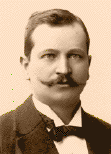Home

Lecture Notes

PHY 251/420:
Introduction to Condensed Matter Physics

Prof. S. Teitel stte@pas.rochester.edu ---- Fall 2006

## Lecture Notes

The lecture note files correspond roughly to the material presented in a given day's lecture. But you may on occassion find the end of one day's lecture at the start of the file for the next day's lecture, so please look there if you think there might be something missing.

Lecture 1 - What is condensed matter physics? The Drude model for d.c. electric conductivity

• Lecture 2 - Hall effect, a.c. conductivity

• Lecture 3 - Electromagnetic wave propagation in a metal, plasma frequency, thermal conductivity and thermoelectric effect

• Lecture 4 - The Sommerfeld model for electrons in a metal, the ideal quantum gas of fermions, ground state of the electron gas, the Fermi energy

• Lecture 5 - The density of states, pressure and bulk modulus of conduction electrons, finite temperature electron gas, specific heat of conduction electrons

• Lecture 6 - Transport properties in the Sommerfeld model, Pauli paramagnetism

• Lecture 7 - Electrons in a uniform magnetic field: Landau levels

• Lecture 8 - Landau diamagnetism

• Lecture 9 - Landau diamagnetism, the de Haas - van Alphen effect

• Lecture 10 - The Integer Quantum Hall Effect
von Klitzing's Nobel Prize Lecture, Rev. Mod. Phys. 58, 519 (1986)
Laughlin's theory paper, Phys. Rev. B 23, 5632 (1981)
Girvin's review article arXiv:cond-mat/9907002
• Lecture 11 - The Integer Quantum Hall Effect continued

• Lecture 12 - The Integer Quantum Hall Effect continued

• Lecture 13 - Screening and the Thomas-Fermi dielectric function

• Lecture 14 - Plasmons, Wigner electron crystal

• Lecture 15 - Cooper pairing

• Lecture 16 - Bravais lattices and crystal structure, lattice with a basis

• Lecture 17 - Reciprocal lattice and X-ray scattering

• Lecture 18 - X-ray scattering continued, Bragg planes

• Lecture 19 - Electrons in a periodic potential - qualitative discussion, Born-von Karman boundary conditions

• Lecture 20 - Fourier transforms on a Bravias lattice, Bloch's theorem, band structure

• Lecture 21 - More on Bloch's theorem, reduced, extended and periodic zone schemes, velocity, weak potential approximation and energy gaps

• Lecture 22 - Ground state, metals, insulators and semiconductors, 2D Brillouin Zones and band structure, geometry of the Fermi surface
The 2D Brillouin Zones in living color!
• Lecture 23 - Semiclassical equations of motion

• Lecture 24 - Motion in uniform electric and magnetic fields, effective mass, holes

• Lecture 25 - Motion in perpendicular electric and magnetic fields, Hall effect with closed orbits

• Lecture 26 - Hall effect with open orbits, real metals

• Lecture 27 - Lattice vibrations, phonons, Bohm-Staver relation and the speed of sound